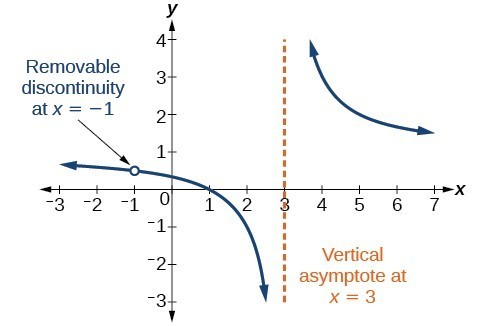## Domain and Its Effect on Vertical Asymptotes

### Learning Outcomes

• Define the domain of a rational function given an equation.
• Use the domain of a rational function to define vertical asymptotes.

A vertical asymptote represents a value at which a rational function is undefined, so that value is not in the domain of the function. A reciprocal function cannot have values in its domain that cause the denominator to equal zero. In general, to find the domain of a rational function, we need to determine which inputs would cause division by zero.

### A General Note: Domain of a Rational Function

The domain of a rational function includes all real numbers except those that cause the denominator to equal zero.

### How To: Given a rational function, find the domain.

1. Set the denominator equal to zero.
2. Solve to find the $x$-values that cause the denominator to equal zero.
3. The domain is all real numbers except those found in Step 2.

### Example: Finding the Domain of a Rational Function

Find the domain of $f\left(x\right)=\dfrac{x+3}{{x}^{2}-9}$.

### Try It

Find the domain of $f\left(x\right)=\dfrac{4x}{5\left(x - 1\right)\left(x - 5\right)}$.

By looking at the graph of a rational function, we can investigate its local behavior and easily see whether there are asymptotes. We may even be able to approximate their location. Even without the graph, however, we can still determine whether a given rational function has any asymptotes, and calculate their location.

Watch the following video to see more examples of finding the domain of a rational function.

## Vertical Asymptotes

The vertical asymptotes of a rational function may be found by examining the factors of the denominator that are not common to the factors in the numerator. Vertical asymptotes occur at the zeros of such factors.

### How To: Given a rational function, identify any vertical asymptotes of its graph.

1. Factor the numerator and denominator.
2. Note any restrictions in the domain of the function.
3. Reduce the expression by canceling common factors in the numerator and the denominator.
4. Note any values that cause the denominator to be zero in this simplified version. These are where the vertical asymptotes occur.
5. Note any restrictions in the domain where asymptotes do not occur. These are removable discontinuities.

### Example: Identifying Vertical Asymptotes

Find the vertical asymptotes of the graph of $k\left(x\right)=\dfrac{5+2{x}^{2}}{2-x-{x}^{2}}$.

## Removable Discontinuities

Occasionally, a graph will contain a hole: a single point where the graph is not defined, indicated by an open circle. We call such a hole a removable discontinuity.

For example, the function $f\left(x\right)=\dfrac{{x}^{2}-1}{{x}^{2}-2x - 3}$ may be re-written by factoring the numerator and the denominator.

$f\left(x\right)=\dfrac{\left(x+1\right)\left(x - 1\right)}{\left(x+1\right)\left(x - 3\right)}$

Notice that $x+1$ is a common factor to the numerator and the denominator. The zero of this factor, $x=-1$, is the location of the removable discontinuity. Notice also that $x - 3$ is not a factor in both the numerator and denominator. The zero of this factor, $x=3$, is the vertical asymptote.### A General Note: Removable Discontinuities of Rational Functions

A removable discontinuity occurs in the graph of a rational function at $x=a$ if a is a zero for a factor in the denominator that is common with a factor in the numerator. We factor the numerator and denominator and check for common factors. If we find any, we set the common factor equal to 0 and solve. This is the location of the removable discontinuity. This is true if the multiplicity of this factor is greater than or equal to that in the denominator. If the multiplicity of this factor is greater in the denominator, then there is still an asymptote at that value.

### Example: Identifying Vertical Asymptotes and Removable Discontinuities for a Graph

Find the vertical asymptotes and removable discontinuities of the graph of $k\left(x\right)=\dfrac{x - 2}{{x}^{2}-4}$.

## Contribute!

Did you have an idea for improving this content? We’d love your input.